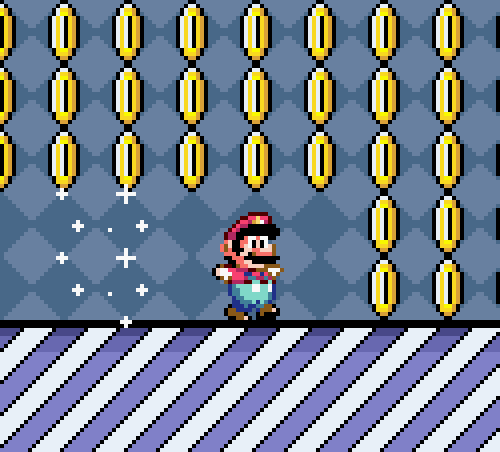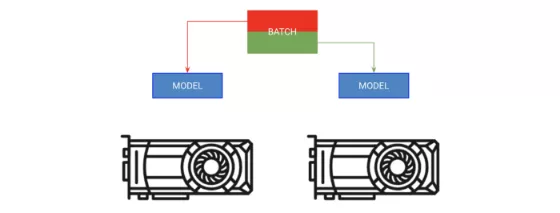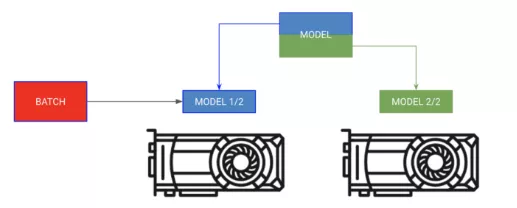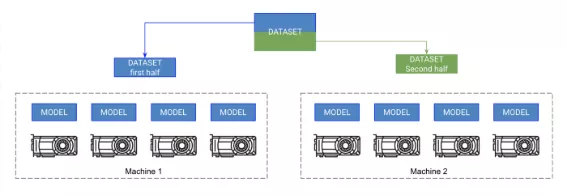# 送你9个快速使用Pytorch训练解决神经网络的技巧（附代码）

+关注继续查看## Pytorch-LightningLightning是基于Pytorch的一个光包装器，它可以帮助研究人员自动训练模型，但关键的模型部件还是由研究人员完全控制。

Lightning采用最新、最尖端的方法，将犯错的可能性降到最低。

from pytorch-lightning import Trainer

model = LightningModule(…)trainer = Trainer()
trainer.fit(model)dataset = MNIST(root=self.hparams.data_root, train=train, download=True)

for batch in loader: x, y = batchmodel.training_step(x, y)...

https://github.com/williamFalcon/pytorch-lightning/blob/master/examples/new_project_templates/lightning_module_template.py?source=post_page---------------------------#L163-L217# slowloader = DataLoader(dataset, batch_size=32, shuffle=True)
# fast (use 10 workers)loader = DataLoader(dataset, batch_size=32, shuffle=True, num_workers=10)

## 3. 批尺寸## 4. 累积梯度# clear last stepoptimizer.zero_grad()

# 16 accumulated gradient stepsscaled_loss = 0for accumulated_step_i in range(16):      out = model.forward()     loss = some_loss(out,y)         loss.backward()

scaled_loss += loss.item()

# update weights after 8 steps. effective batch = 8*16optimizer.step()

# loss is now scaled up by the number of accumulated batchesactual_loss = scaled_loss / 16

trainer.fit(model)

## 5. 保留计算图losses = []
...losses.append(loss)

print(f'current loss: {torch.mean(losses)'})

# badlosses.append(loss)

# goodlosses.append(loss.item())

Lightning会特别注意，让其无法保留图形副本

## 6. 转至单GPU# put model on GPUmodel.cuda(0)

# put data on gpu (cuda on a variable returns a cuda copy)x = x.cuda(0)

# runs on GPU nowmodel(x)

# ask lightning to use gpu 0 for trainingtrainer = Trainer(gpus=)trainer.fit(model)

# expensivex = x.cuda(0)

# very expensivex = x.cpu()x = x.cuda(0)


# really bad idea.Stops all the GPUs until they all catch uptorch.cuda.empty_cache()


## 7. 16位混合精度训练

16位精度可以有效地削减一半的内存占用。大多数模型都是用32位精度数进行训练的。然而最近的研究发现，使用16位精度，模型也可以很好地工作。混合精度指的是，用16位训练一些特定的模型，而权值类的用32位训练。

# enable 16-bit on the model and the optimizermodel, optimizers = amp.initialize(model, optimizers, opt_level='O2')
# when doing .backward, let amp do it so it can scale the losswith amp.scale_loss(loss, optimizer) as scaled_loss:                           scaled_loss.backward()

amp包会处理大部分事情。如果梯度爆炸或趋于零，它甚至会扩大loss。

trainer = Trainer(amp_level=’O2', use_amp=False)trainer.fit(model)

## 8. 移至多GPUA在每个GPU上复制模型；B给每个GPU分配一部分批量。

# copy model on each GPU and give a fourth of the batch to eachmodel = DataParallel(model, devices=[0, 1, 2 ,3])

# out has 4 outputs (one for each gpu)out = model(x.cuda(0))


# ask lightning to use 4 GPUs for trainingtrainer = Trainer(gpus=[0, 1, 2, 3])trainer.fit(model)# each model is sooo big we can't fit both in memory
encoder_rnn.cuda(0)
decoder_rnn.cuda(1)

# run input through encoder on GPU 0
out = encoder_rnn(x.cuda(0))

# run output through decoder on the next GPU
out = decoder_rnn(x.cuda(1))

# normally we want to bring all outputs back to GPU 0
out = out.cuda(0)



class MyModule(LightningModule):

def __init__():         self.encoder = RNN(...)        self.decoder = RNN(...)

def forward(x):

# models won't be moved after the first forward because         # they are already on the correct GPUs        self.encoder.cuda(0)        self.decoder.cuda(1)        out = self.encoder(x)        out = self.decoder(out.cuda(1))

# don't pass GPUs to trainermodel = MyModule()trainer = Trainer()trainer.fit(model)

# change these linesself.encoder = RNN(...)self.decoder = RNN(...)

# to these# now each RNN is based on a different gpu setself.encoder = DataParallel(self.encoder, devices=[0, 1, 2, 3])self.decoder = DataParallel(self.encoder, devices=[4, 5, 6, 7])

# in forward...out = self.encoder(x.cuda(0))

# notice inputs on first gpu in devicesout = self.decoder(out.cuda(4))  # <--- the 4 here

## 9. 转至多GPU阶段（8+GPUs）Pytorch在各个GPU上跨节点复制模型并同步梯度，从而实现多节点训练。因此，每个模型都是在各GPU上独立初始化的，本质上是在数据的一个分区上独立训练的，只是它们都接收来自所有模型的梯度更新。

On .backward() 所有副本都会接收各模型梯度的副本。只有此时，模型之间才会相互通信。

Pytorch有一个很好的抽象概念，叫做分布式数据并行处理，它可以为你完成这一操作。要使用DDP（分布式数据并行处理），需要做4件事：

def tng_dataloader():     d = MNIST()

# 4: Add distributed sampler     # sampler sends a portion of tng data to each machine     dist_sampler = DistributedSampler(dataset)     dataloader = DataLoader(d, shuffle=False, sampler=dist_sampler)

def main_process_entrypoint(gpu_nb):      # 2: set up connections  between all gpus across all machines     # all gpus connect to a single GPU "root"     # the default uses env://

world = nb_gpus * nb_nodes     dist.init_process_group("nccl", rank=gpu_nb, world_size=world)

# 3: wrap model in DPP     torch.cuda.set_device(gpu_nb)     model.cuda(gpu_nb)     model = DistributedDataParallel(model, device_ids=[gpu_nb])

if  __name__ == '__main__':      # 1: spawn number of processes     # your cluster will call main for each machine     mp.spawn(main_process_entrypoint, nprocs=8)



# train on 1024 gpus across 128 nodes
trainer = Trainer(nb_gpu_nodes=128, gpus=[0, 1, 2, 3, 4, 5, 6, 7])


Lightning还附带了一个SlurmCluster管理器，可助你简单地提交SLURM任务的正确细节。


# train on 4 gpus on the same machine MUCH faster than DataParallel
trainer = Trainer(distributed_backend='ddp', gpus=[0, 1, 2, 3])


## 10. 有关模型加速的思考和技巧|
2月前
|

【深度学习】实验10 使用Keras完成逻辑回归
【深度学习】实验10 使用Keras完成逻辑回归
21 0
|
6月前
|

PyTorch神经网络
PyTorch神经网络
26 0
|
6月前
|

pytorch实现前馈神经网络实验（手动实现）
pytorch实现前馈神经网络实验（手动实现）
111 0
|
7月前
|

【Pytorch神经网络实战案例】33 使用BERT模型实现完形填空任务

259 0
|
7月前
|

【Pytorch神经网络理论篇】 39 Transformers库中的BERTology系列模型

98 0
|
7月前
|

109 0
|
7月前
|

【Pytorch神经网络实战案例】05 使用Pytorch完成Logistic分类
【Pytorch神经网络实战案例】05 使用Pytorch完成Logistic分类
43 0
|
7月前
|

【Pytorch神经网络实战案例】04 使用Pytorch实现线性回归
【Pytorch神经网络实战案例】04 使用Pytorch实现线性回归
72 0